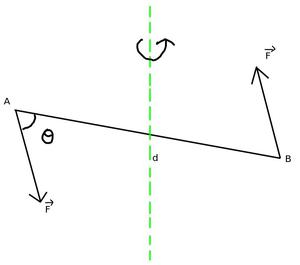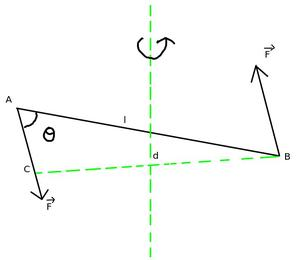GFG App
Open AppBrowser
Continue

# What are Couples? Definition, Moment of Couple, Applications

A pair of hand forces spin an automobile’s steering wheel, while a screwdriver is twisted by the equivalent of a couple. A wrench, on the other hand, is operated by a force applied at just one end, resulting in an imbalanced force on the element being tightened in addition to the turning moment.

In mechanics, a couple is a pair of equal parallel forces that are pointing in opposing directions. A couple’s only impact is to cause or inhibit the turning of a body. The turning effect, or moment, of a pair, is calculated by multiplying the magnitude of either forces by the perpendicular distance between their action lines.

When a force is applied to make a body rotate, according to Newton’s third law, an equal and opposite reaction force is exerted on the point about which the body rotates (because the forces are always applied in pairs), but this reaction force is the moment of a force about the point of rotation is zero because the perpendicular distance of the reaction force from the point of rotation is zero. As a result, the body rotates only under the influence of torque, τ = F × r. To increase the rotational effect, two equal and opposite forces F can be applied simultaneously on the body at two different points A and B.

### Couple

When two equal and opposite forces are applied simultaneously at different points on a body, their resultant force is zero, but both these equal and opposite forces try to rotate the body in the same direction. These forces are called Couple.

Such a force pair has a tendency to rotate that body. Consider A and B as the two points on a body on which two forces F of the equal result are applied in opposite directions parallel to each other. Due to the effect of this force, this body starts rotating in the anti-clockwise direction or tries to rotate about the axis passing through 0. Keep in mind that if the line of action of these two equal forces is the same or they act at the same point, then they will cancel each other.

A torque operates on a body as a result of a couple’s activity, causing the body to spin around a fixed point.

τ = F × r

which denotes the torque applied on the body. The magnitude of torque equals the quantity of force multiplied by the perpendicular distance between the two forces.

Characteristics of the Couples

1. As the two forces that make up the couple are equal and opposing, the couple does not create translational motion.
2. When it operates on a body, the net resulting force on the body is zero.
3. Since, the algebraic sum of the moments of the two forces around any point in their plane is not zero, it causes pure rotational motion in the body.
4. A couple’s moment about any point in its plane is constant in size and direction.

### Moment of the Couple

The tendency of a force is to rotate a body. It is measured by the moment of the force. The product of one of the two forces of a Couple and the perpendicular distance between their lines of action (called the arm of the Couple) is called the Moment of Couple

Mathematically, the moment of the couple is defined as the product of the force and the perpendicular distance between the lines of action of the two forces. Here, the perpendicular distance between the lines of action of two forces is also called the arm of the Couple. That is the moment of force is also equal to the product of the applied force and the arm of a pair of forces.

Thus, the moment of pair of forces is given by,

τ  = F × d                                                                                                                                                                                                     …….(1)It is clear from the formula for the moment of a pair of forces that the moment of a pair of forces will be greater if

• The magnitude of the force is greater, and
• The arm of the pair of forces is longer, i.e. the perpendicular distance between the lines of action of the two forces is greater.

The SI unit of Moment of Couple is Newton-Meter (N m). And the dimensional formula of Moment of Couple is [ML2T-2].

Types of Moment of Couple

There are two types of moments of couple, that are:

1. Positive moment: When the body tends to rotate in the anticlockwise direction under the influence of a Couple, then its moment is called positive moment
2. Negative moment: When the force pair tends to rotate in the clockwise direction, then its moment is called negative moment.

### Vector form of Moment of Couple

Lets draw BC perpendicular to the line of action of the force F acting from point B to A. Therefore, ∠BAC = θ.And, τ  = F × BC

But from right angle ΔABC: sin θ = BC / AB,

or

BC = AB sin θ

Now, putting values in equation (1),

τ  = F × AB sin θ

or

τ  = F × l sin θ                                                                                                                                                                                        …….(2)

= l × F sin θ

τ  = l ×  F

This is the expression of Moment of Couple in vector form, where l = AB

### Practical Application of Couples

1. When turning a moving bicycle, we apply force to the handle with our hands.
2. When we open and close the spout of the tap.
3. While opening and closing the lid of the device, a pair of forces is applied with the fingers.
4. Keeping the pencil between the palms of both hands, the pencil starts rotating when the palms are run in the opposite direction.
5. While holding the ends of the rope with the hand while running the hand in the opposite direction, the churn starts rotating, this is also an example of a couple.

### Sample Problems

Problem 1: A rope wrapped around a hollow cylinder of radius 40 cm is pulled by applying a force of 30 N. find the torque exerted on the cylinder.

Solution:

Given that,

F = 30 N,

r = 40 cm = 0.4 m.

Moment of Couple applied on the hollow, τ = Fr

= 30 × 0.4

= 12 Nm

Problem 2: A body is riveted at some point. a force of magnitude 10 N is applied on the body at a distance of 40 cm from this point. what will be the moment of a force about the riveted point?

Solution:

Given that,

r = 40 cm = 0.4 m and

F = 10 N.

Therefore, Moment of Couple about the riveted point is:

τ = Fr

= 10 × 0.4

= 4 Nm

Problem 3: The diameter of the steering wheel of a car is 40 cm. If the driver turns the wheel by applying two equal and opposite forces of 0.1 N on the diametrical ends of the wheel, calculate the torque exerted on the wheel.

Solution:

A force of a pair of forces, F = 0.1 N.

Side of a pair of forces = diameter of a wheel = 40 cm = 0.4 m.

Moment of Couple = One force of a pair of forces × side pair of forces

= 0.1 × 0.4 = 0.04 Nm

Problem 4: To keep the angular speed of rotation of a wheel constant at 200 rad/s, a torque of 180 Nm has to be applied by the engine. Find the power required by the engine. Engine efficiency is 100%.

Solution:

It is clear that in order to keep the angular speed of rotation of the wheel uniform, the moment of force is required to be applied to cancel the moment of friction.

Required power of the engine, P = Torque × Angular speed

= 180 × 200

= 36000 W

Problem 5: How many newtons of force should be applied 50 cm away from the hinge of a door so that the value of the torque is 5 Nm.

Solution:

Given that,

r = 50cm = 0.5 m and

τ =  5 Nm

Using the formula mentioned below,

τ = F × r

Substituting the given values in the above expression as,

5 Nm = F × 0.5 m

or

F = 5 Nm / 0.5 m

= 10 N

My Personal Notes arrow_drop_up